# E-PolyLearning

 56. Choose the figure which is different from the rest.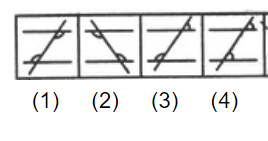a. 1 b. 2 c. 3 d. 4

 57. Choose the figure which is different from the rest.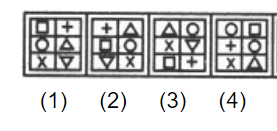a. 1 b. 2 c. 3 d. 4
 58. Choose the figure which is different from the rest.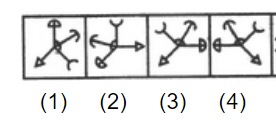a. 1 b. 2 c. 3 d. 4
 59. Choose the figure which is different from the rest.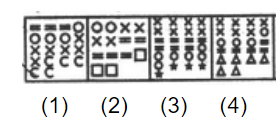a. 1 b. 2 c. 3 d. 4
 60. Choose the figure which is different from the rest.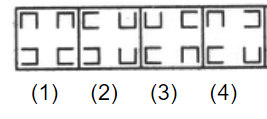a. 1 b. 2 c. 3 d. 4